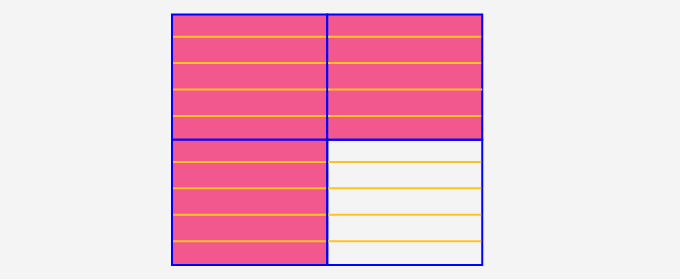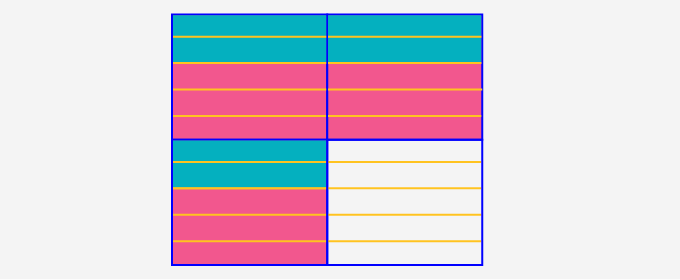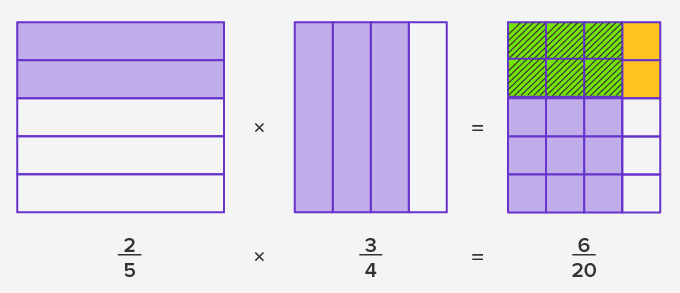# Multiplying Fractions - Definition with Examples

The Complete K-5 Math Learning Program Built for Your Child

• 40 Million Kids

Loved by kids and parent worldwide

• 50,000 Schools

Trusted by teachers across schools

• Comprehensive Curriculum

Aligned to Common Core

## Multiplying Fractions

A fraction is a part of a whole.An apple pie cut into 4 equal slices and one slice is kept apart as shown.

Here, the apple pie is cut into 4 equal parts and each part represents one-fourth of the pie. How much apple pie would be there in 5 such pieces?

It would be the product of 5 × 1 4 . We can evaluate the multiplication as repeated addition also, and it is easier.

5 × 1 4 = 1 4 + 1 4 + 1 4 + 1 4 + 1 4 = 5 4

We can also convert this into a mixed number, 5 4  = 1 1 4 . Therefore, 5 pieces of the pie will have one and a quarter of apple pie.

But the repeated addition is not always an easier method, especially when the multiplier is also a fraction.

Consider the product 2 5 × 3 4 .

The fraction 3 4 can be represented as shown:Now, the required product is the two-fifth of this shaded part.

To find that, you need to divide these three shaded part into 5 equal parts. An easier way to do this is to divide each of these 4 parts into 5 equal parts.Now, the two-fifths of the three-fourths are the two shaded parts from each of these three parts, that is, 6 shaded parts out of 20 as shown.Another way of representing this geometrically is:In the fraction representing the product, the whole is divided into 20 equal parts and the shaded parts common to both the factors is the denominator, and 6 represents the numerator of the product.

## Algebraically the rule to multiply two fractions is:

Step 1: Multiply the numerators of the factor fractions.

Step 2: Multiply the denominators.

Step 3: Simplify the product if required.

Example:

5 6 x 3 8 = 5 × 3 6 × 8 = 15 48

Here, 3 is a common factor of the numerator and the denominator. So, to simplify the fraction, divide both numerator and denominator by 3.

15 ÷ 3 48 ÷ 3 = 5 6

Thus, 5 6 x 3 8 = 5 16 .

The rule is:

 If a b and c d are fractions with b, d ≠ 0, then a b x c d = ac bd

 Fun Facts The word fraction comes from the Latin word ‘fractio’ which means to break. When two fractions are multiplied, if one of the fractions is greater than 1, it will increase the size of the second fraction as the product. If it is less than 1, it will decrease the size of the second fraction as the product.

Won Numerous Awards & Honors